# IR infrared sensor with Arduino – How does work IR infrared sensor.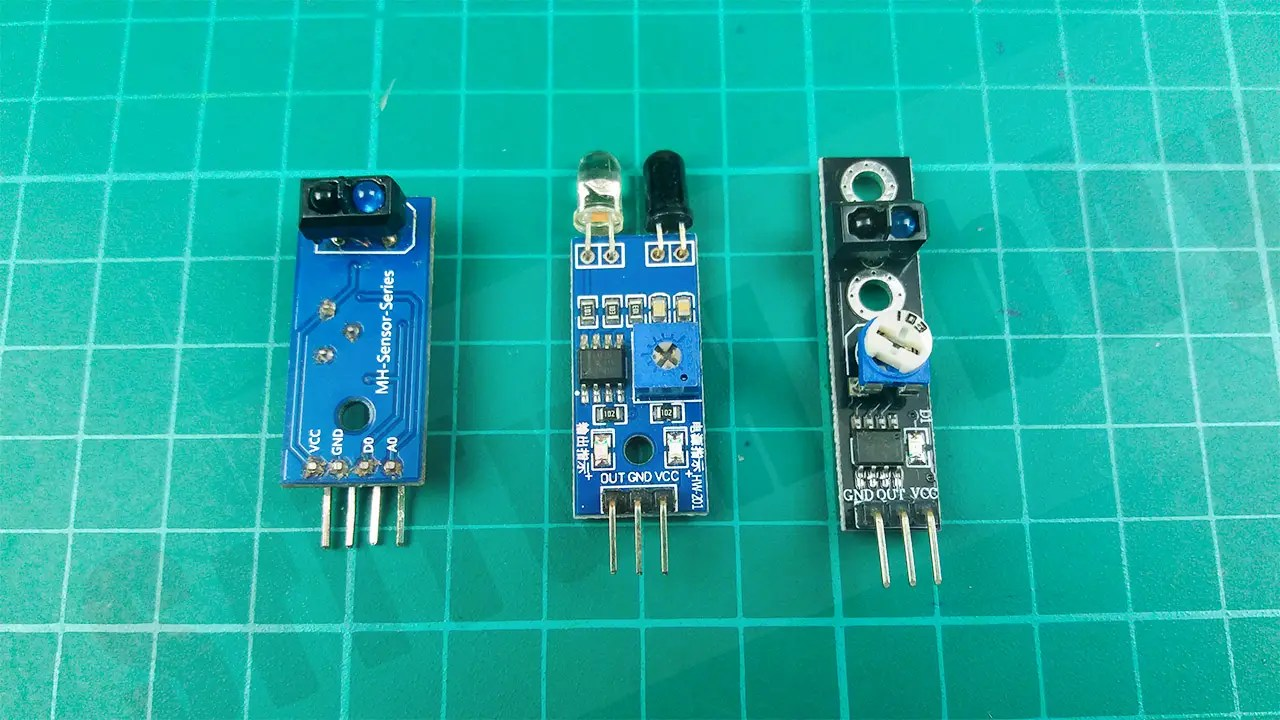Hello guys, Welcome back to my blog. We described the PIR(Motion) sensor in the previous post. Today we are going to talk about the IR sensor modules in this post. This sensor is the most important for making robots. I promise to present those projects in the next articles.

### What is the IR sensor?

An IR sensor is a sensor that can take values ​​using IR rays. This is done by reflecting IR rays. It includes two IR LEDs.One IR LED emits IR rays while the other IR LEDs receive IR rays. These IR rays are invisible to our eyes. If you want to see these IR rays, look through a camera. These IR sensors can be used for two functions. It is used to identify black and white and also to identify an obstacle. This sensor is mainly used to make line-following robots. These sensors have a reflection distance of 1mm to 25mm and up to 30 degrees. We can change this distance using the preset controller. There are different types of these sensors in the market. But both of these sensors do the same thing. Also, these sensors are available to you at very low prices in the market. Let’s do projects using these three types of sensors separately. Below are the components required for this project and the purchase links.

### 1st Sensor type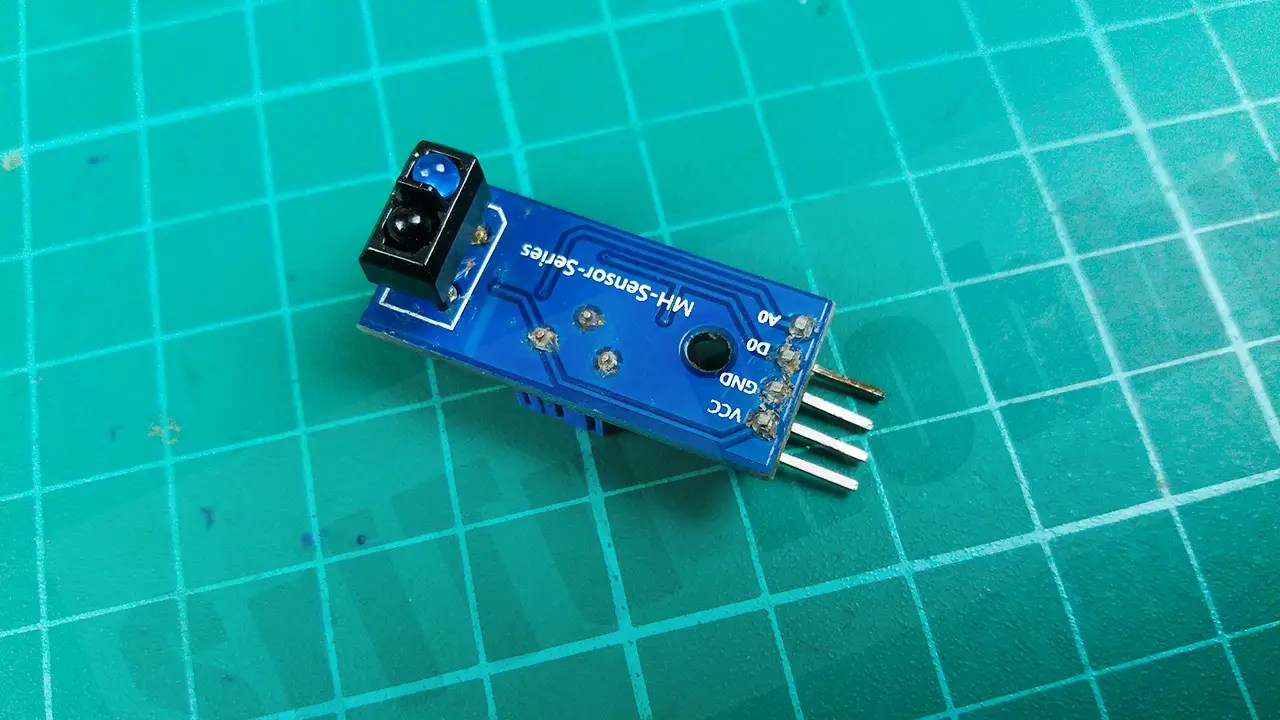This sensor has four pins. The DO pin of the sensor returns a digital value and the AO pin returns an analog value. If you want to change the sensing range, do so with the preset control.

#### PIN structure of this sensor.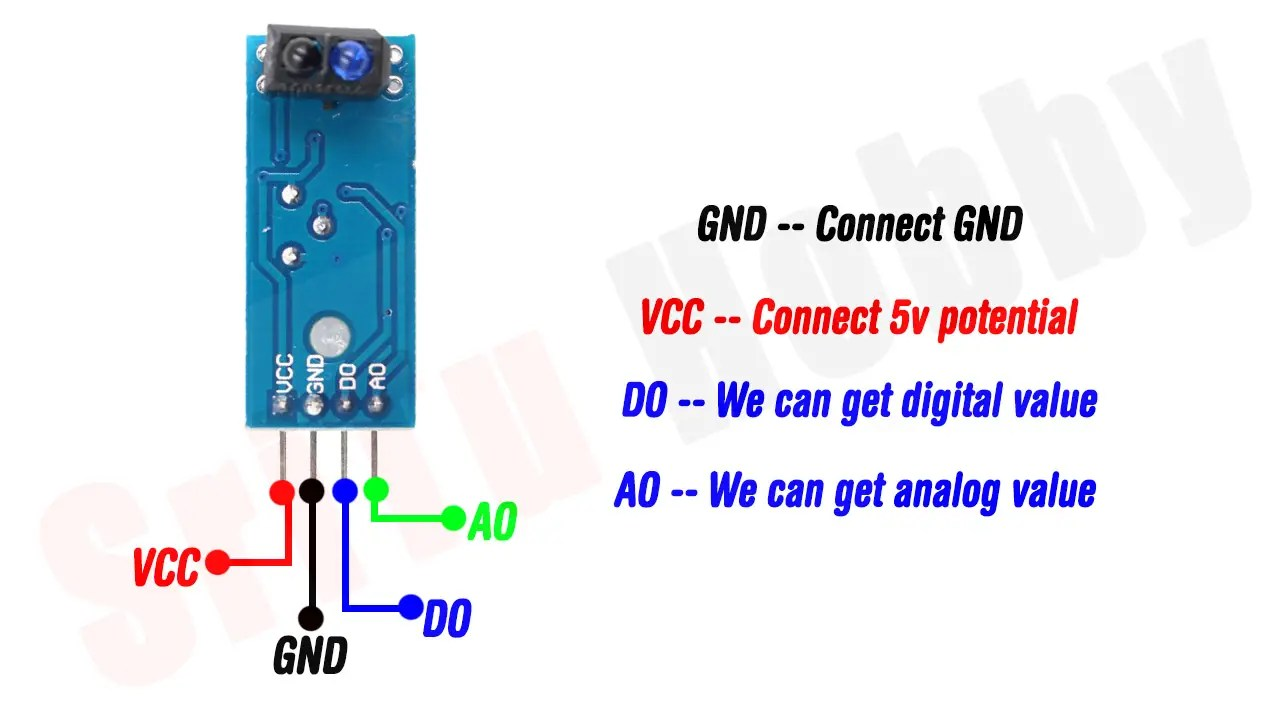### Components

Step 1

Let us identify these components one by one.
Arduino Uno boardYou can use any other board for this project.
IR sensor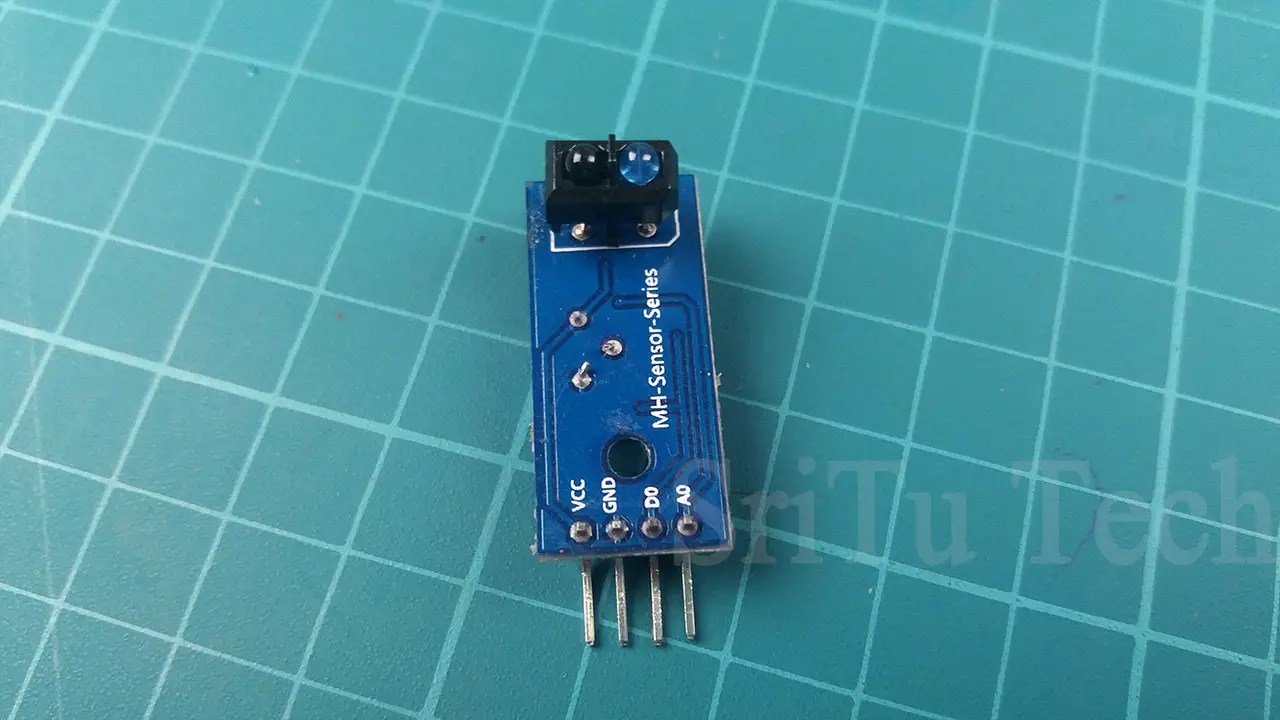LED bulbsBuzzer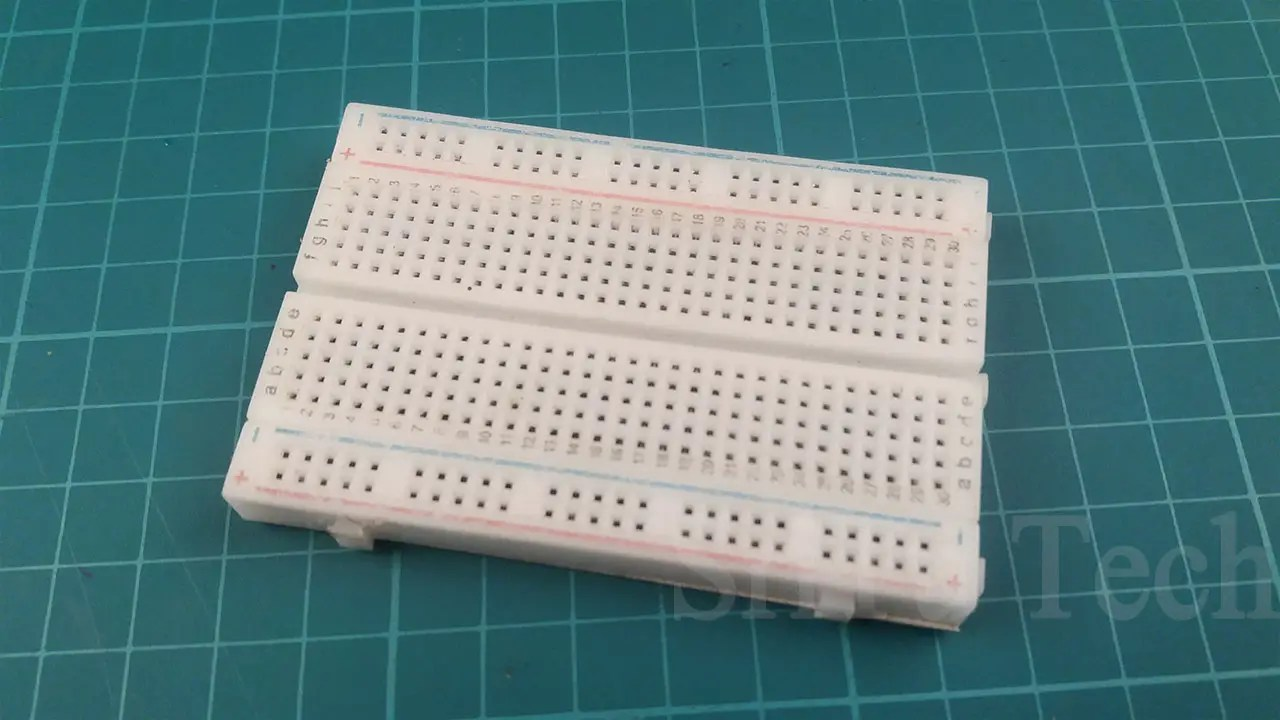Use this component to assemble the circuit.
Jumper wires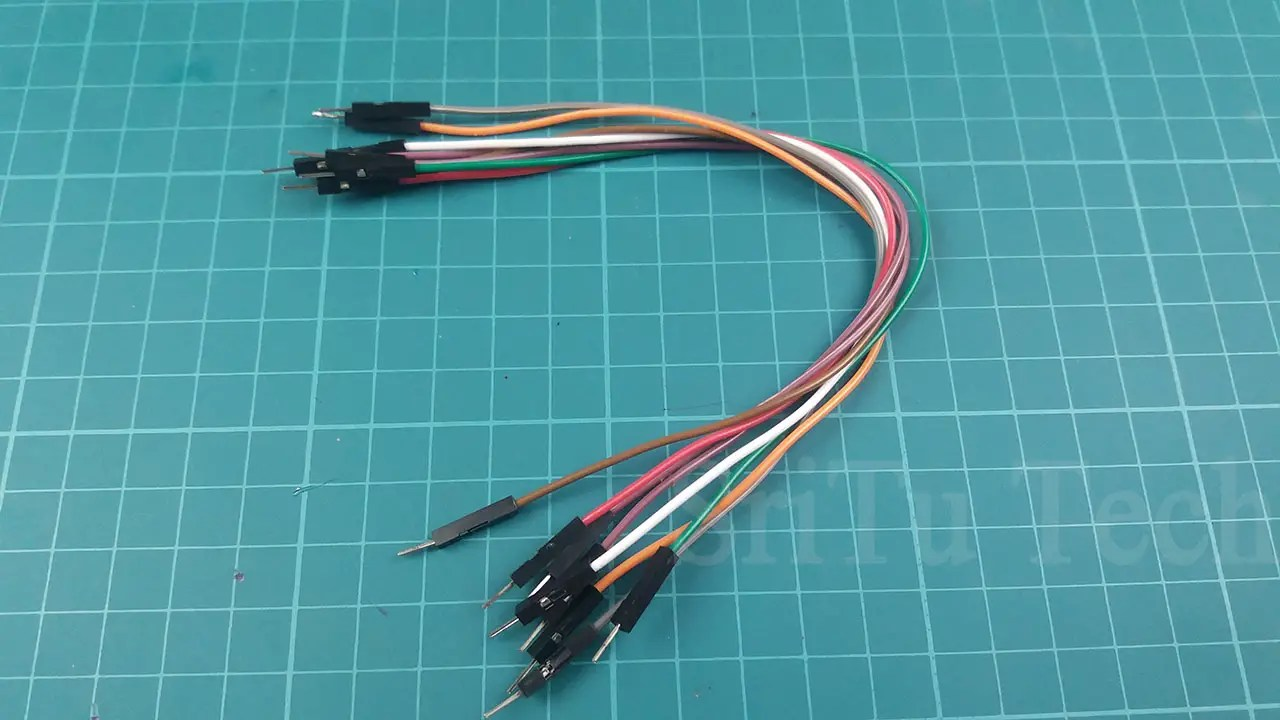Eight jumper wires from MALE to MALE have been used in this project.

Step 2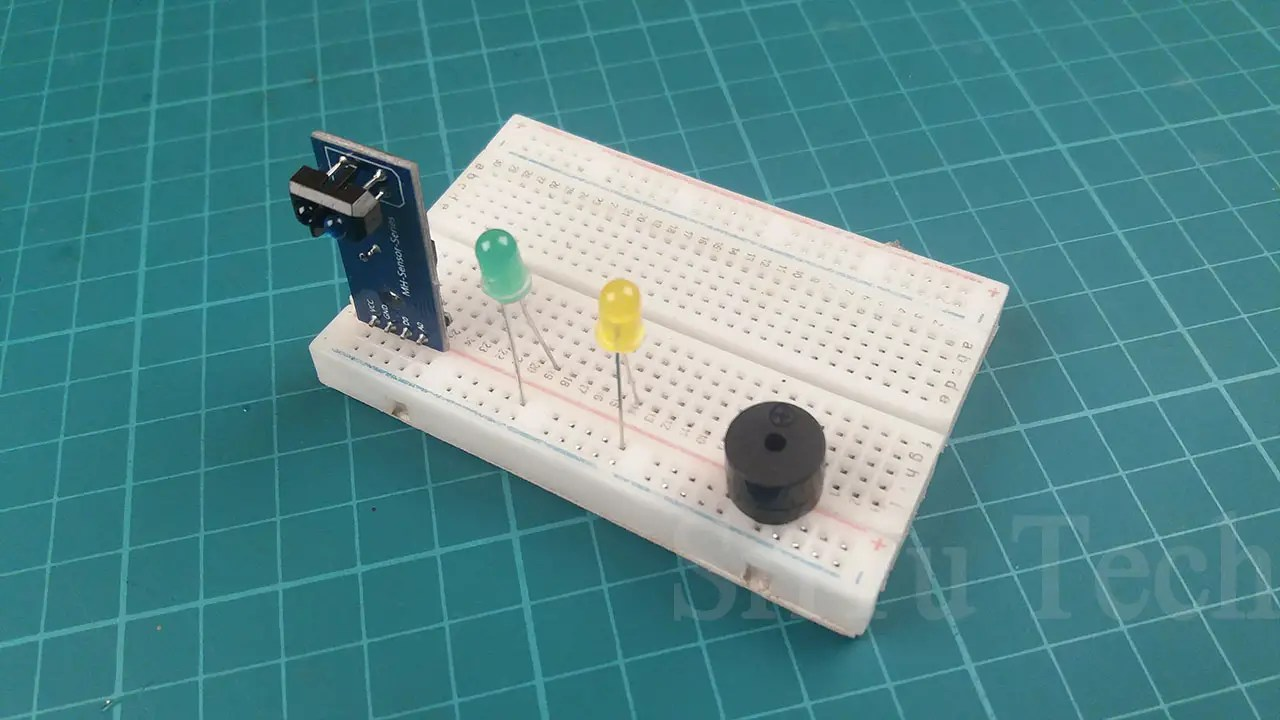Step 3

Connect this circuit to the Arduino Uno board. Use the following circuit diagram. Use jumper wires for it.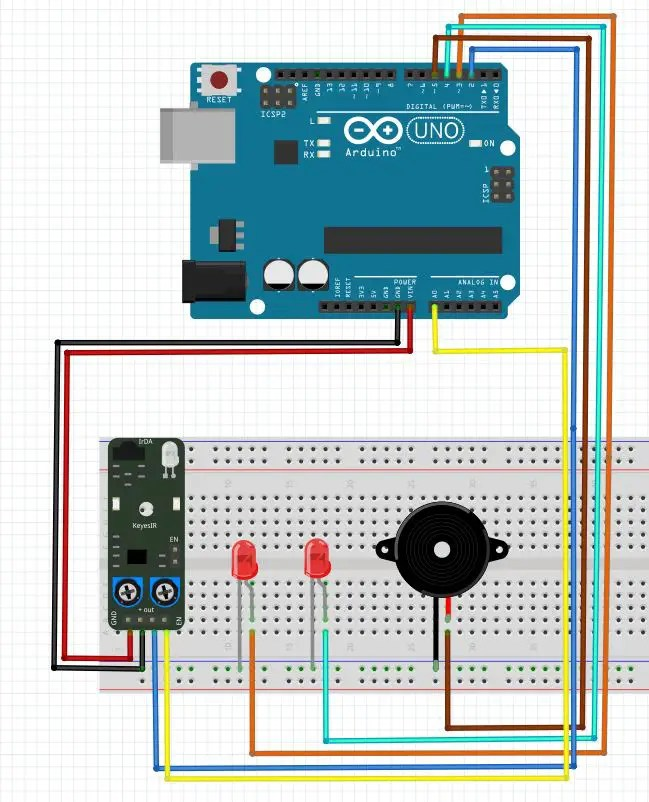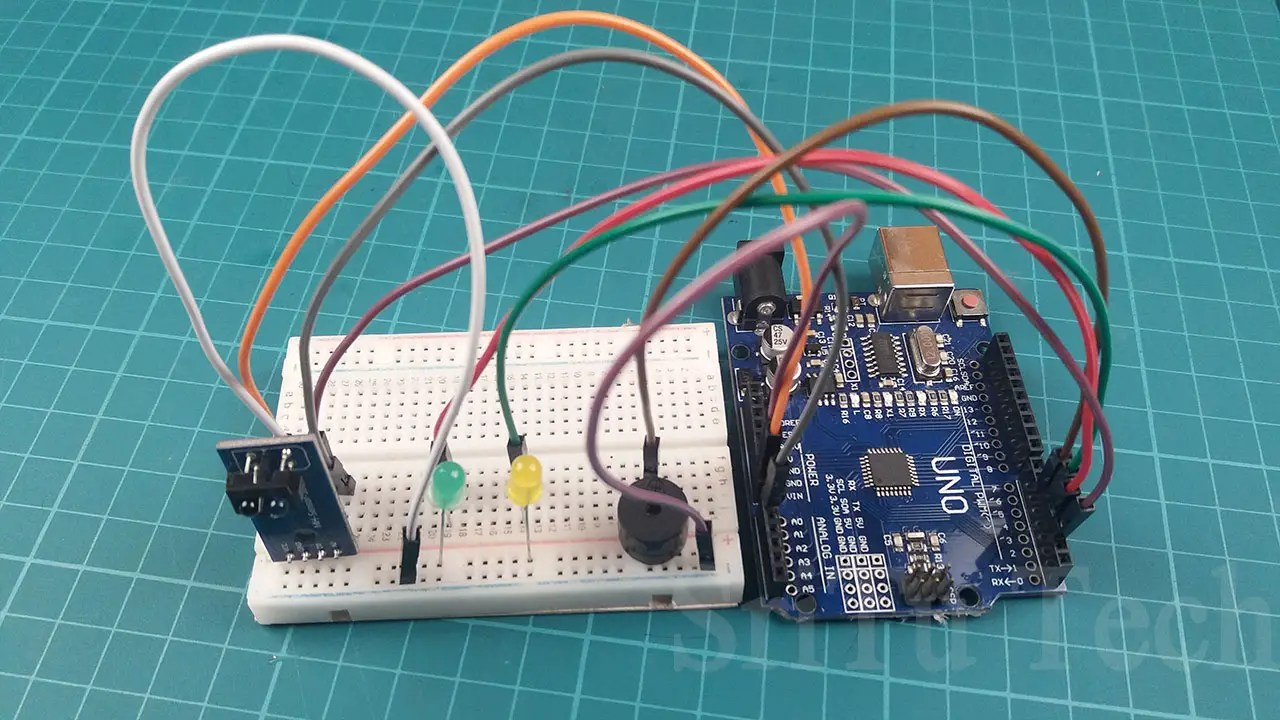The DO pin of the IR sensor connects the D2 pin on the Arduino board and the AO pin connects the A0 pin on the Arduino board. Connect the two LED bulbs and the buzzer with the D2, D3, and D4 pins on the Arduino board. Connect all cathode pins to the GND pin on the Arduino.
Step 4
Look at the code below.
``````void setup() {
Serial.begin(9600);//enable serial monitor
pinMode(2, INPUT);//define arduino pin
pinMode(3, OUTPUT);//define arduino pin
pinMode(4, OUTPUT);//define arduino pin
pinMode(5, OUTPUT);//define arduino pin
}

void loop() {
balckwhite();//Identification of black and white
obstacle();//Identification obstacles.

}
void balckwhite() {
if (led == 0) {
Serial.println("White");
digitalWrite(3, HIGH);
digitalWrite(4, LOW);
} else {
Serial.println("Black");
digitalWrite(4, HIGH);
digitalWrite(3, LOW);
}
}
void obstacle() {
if (buzzer <= 80) {
digitalWrite(5, HIGH);
Serial.println("Buzzer on");
} else {
digitalWrite(5, LOW);
Serial.println("Buzzer off");
}
}``````
This code sets the 2nd pin as the input pin and the 3,4,5 pin as the output pin.
void setup() {
Serial.begin(9600);//enable serial monitor
pinMode(2, INPUT);//define arduino pin
pinMode(3, OUTPUT);//define arduino pin
pinMode(4, OUTPUT);//define arduino pin
pinMode(5, OUTPUT);//define arduino pin
}
This code takes the digital value and puts it into the Boolean variable. The Boolean variable is named ‘led’. This value is checked using the IF condition. When the digital value is 0, the green LED  bulb turns on and when the digital value is 1, the yellow LED bulb turns on.
void balckwhite() {
if (led == 0) {
Serial.println(“White”);
digitalWrite(3, HIGH);
digitalWrite(4, LOW);
} else {
Serial.println(“Black”);
digitalWrite(4, HIGH);
digitalWrite(3, LOW);
}
}
This code takes the analog value and it put into the integer variable. The integer variable is named “buzzer”. This value is checked using the IF condition. If the analog value is less than or equal to 80, the buzzer turns on.
void obstacle() {
if (buzzer <= 80) {
digitalWrite(5, HIGH);
Serial.println(“Buzzer on”);
} else {
digitalWrite(5, LOW);
Serial.println(“Buzzer off”);
}
}
You can view this on a serial monitor.

Step 5
Select board and port.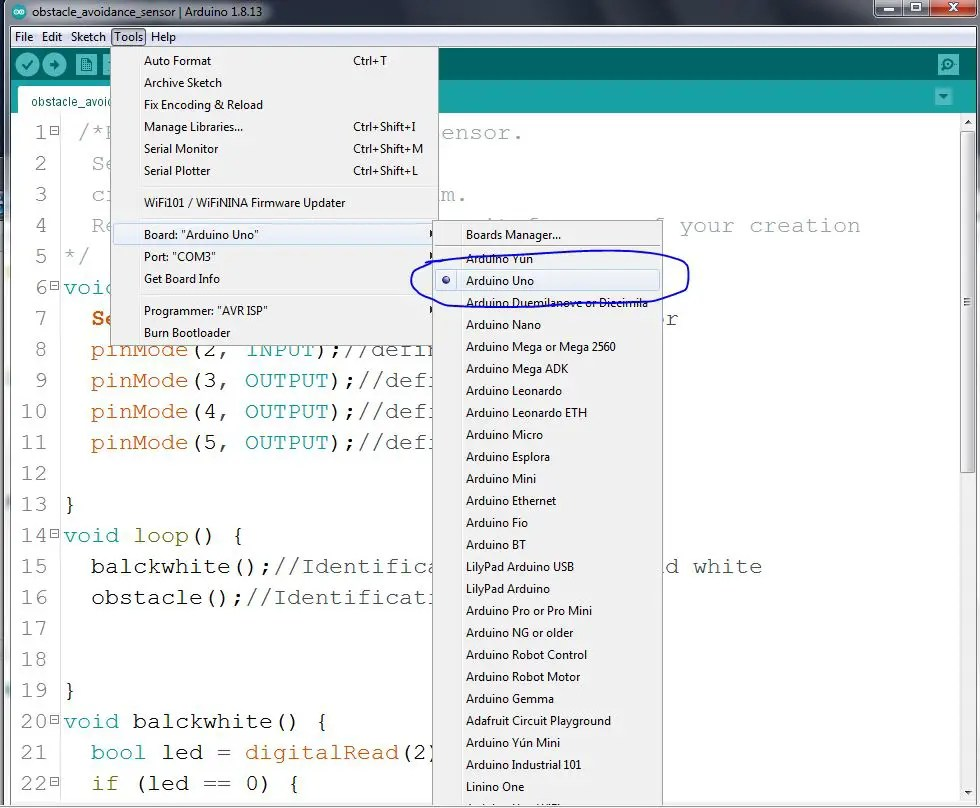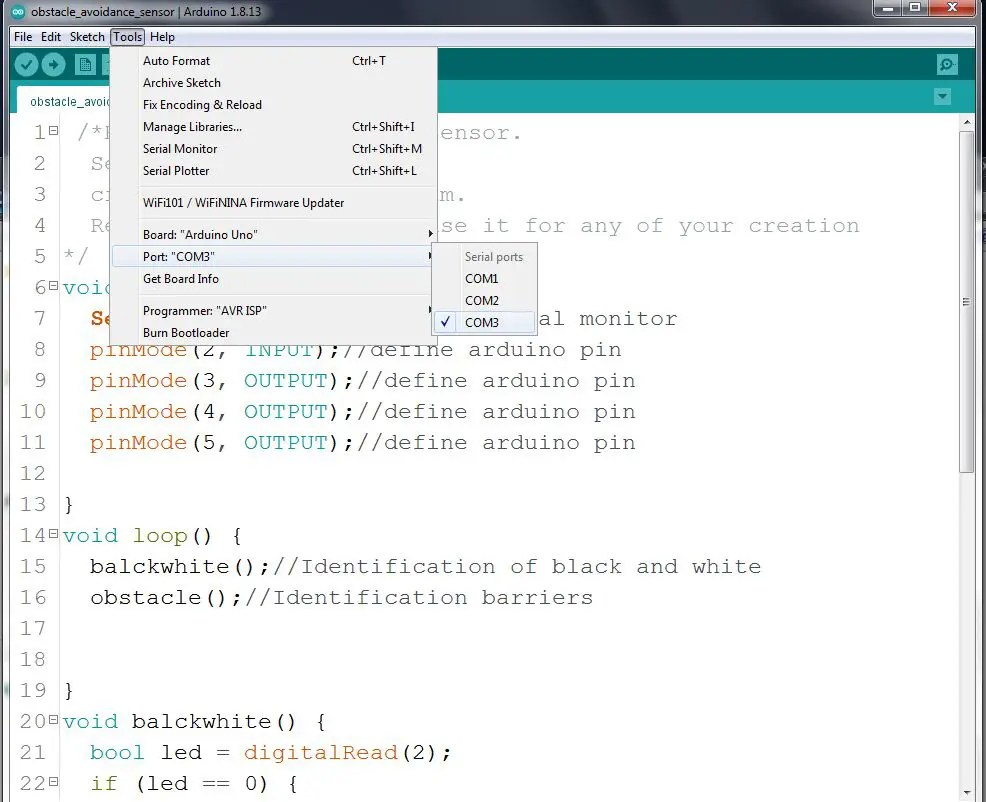Step 6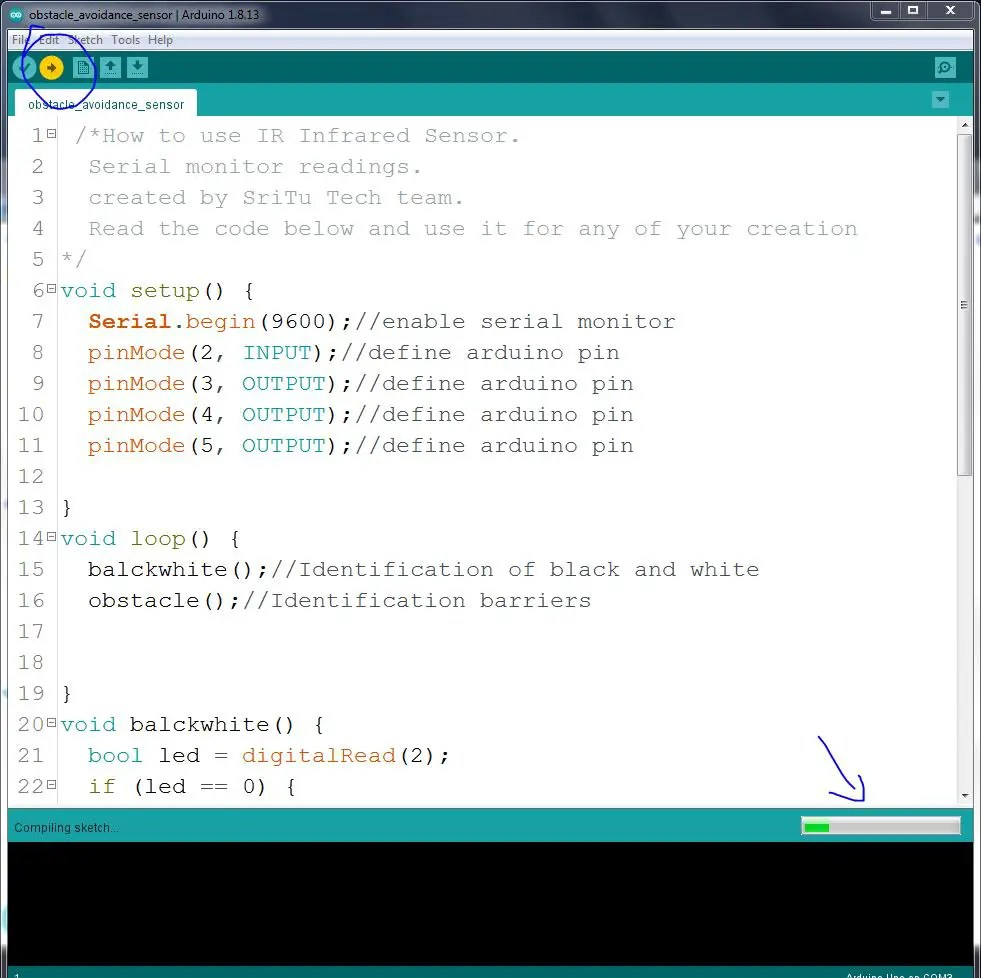You can test it using a black and white surface. The full video guide is given below.

### 2nd sensor type

Okay, let’s see how the other IR sensors are used. It has three pins. The components required for this project are as follows. In this project, we have only talked about identifying the obstacle.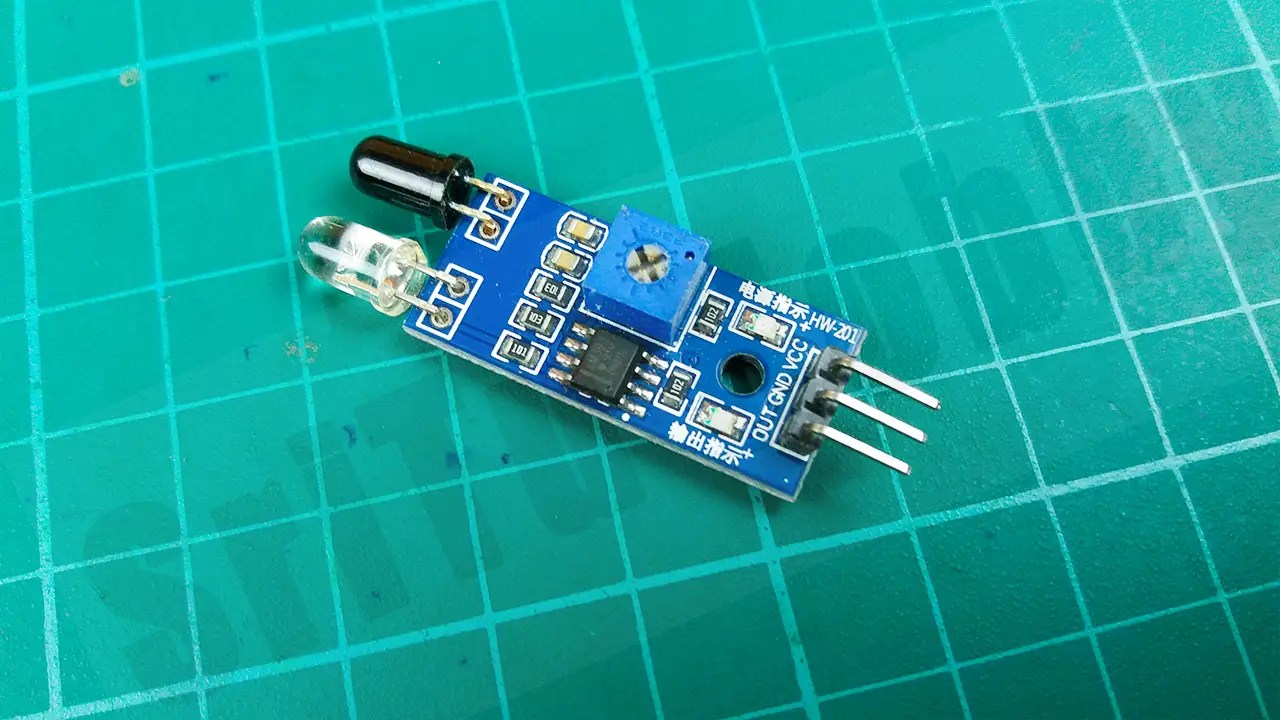#### PIN structure of this sensor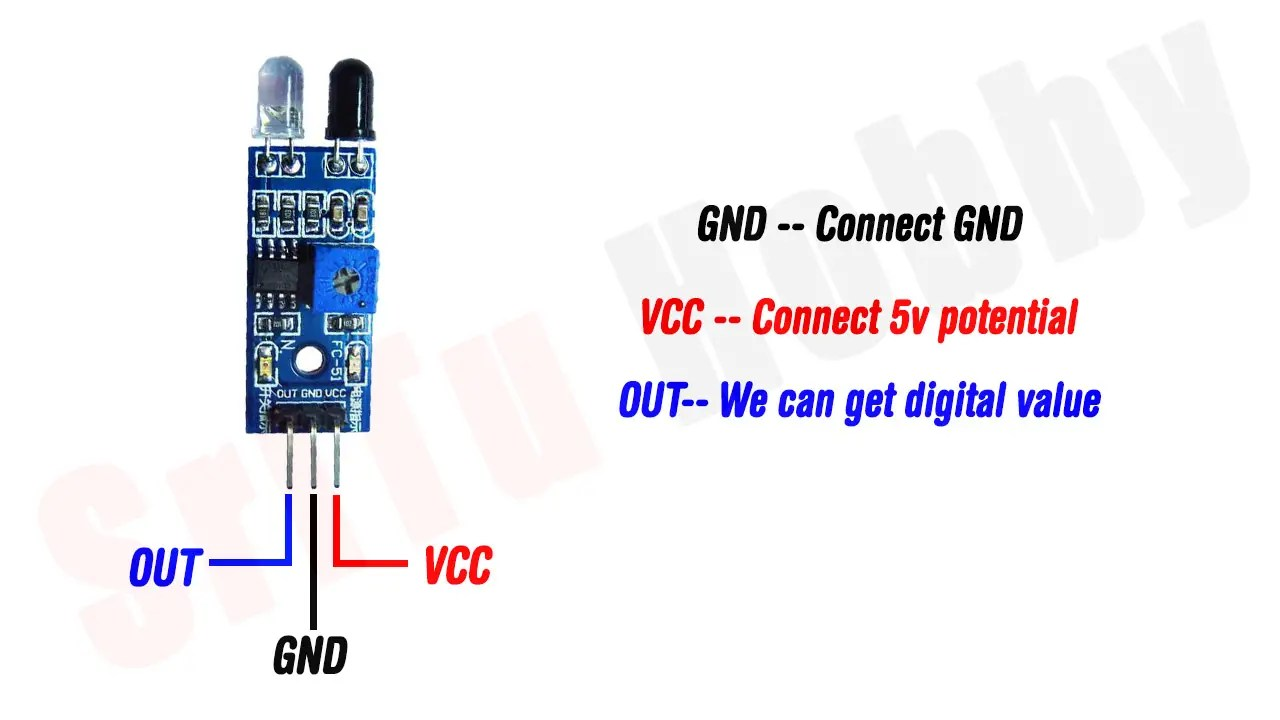So, step by step we will learn how to connect this sensor with Arduino. For that, the required components are given below.

### Components

Step 1

We identify the above components.
Arduino Nano board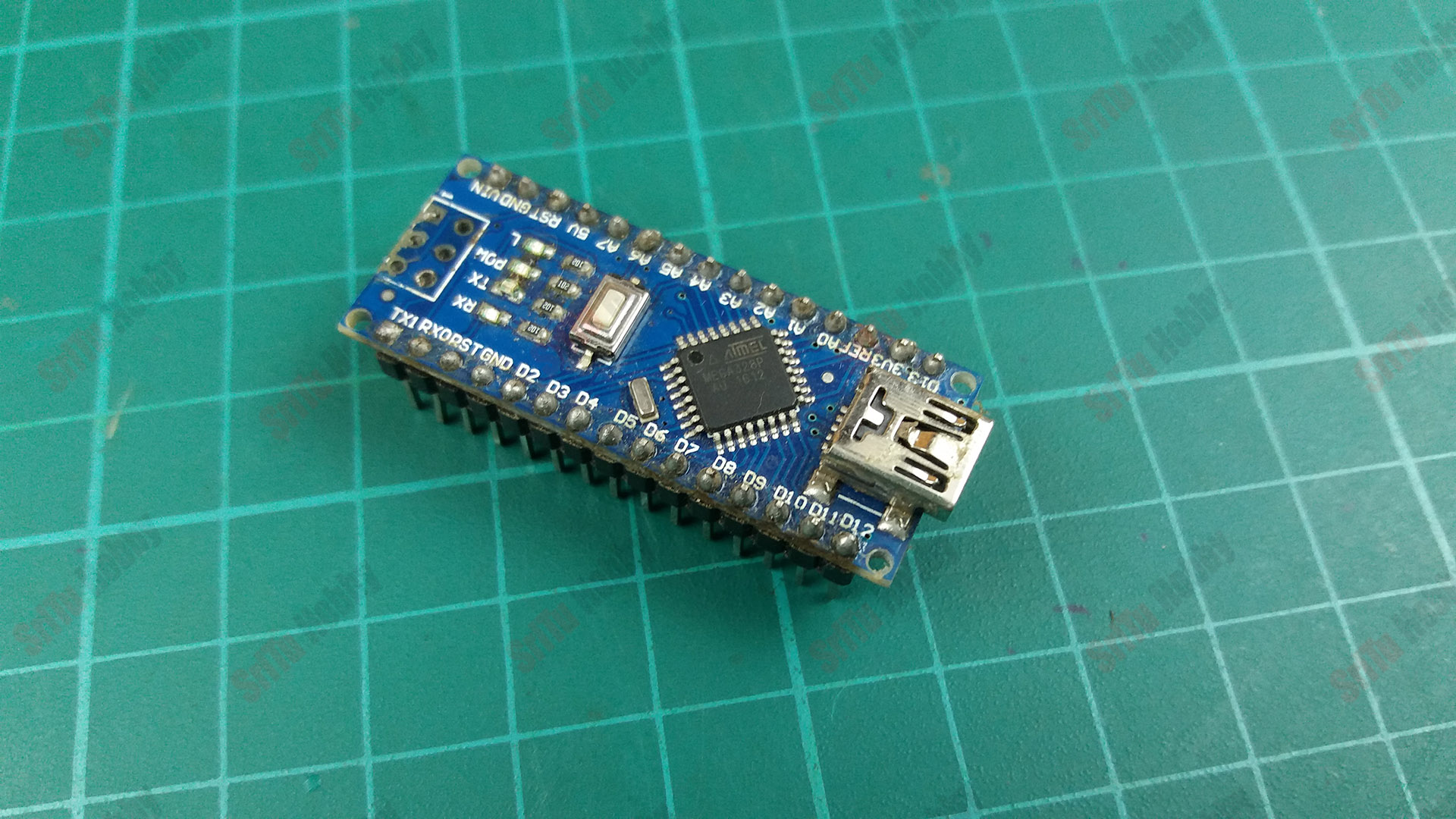You can use any other Arduino board.
IR sensor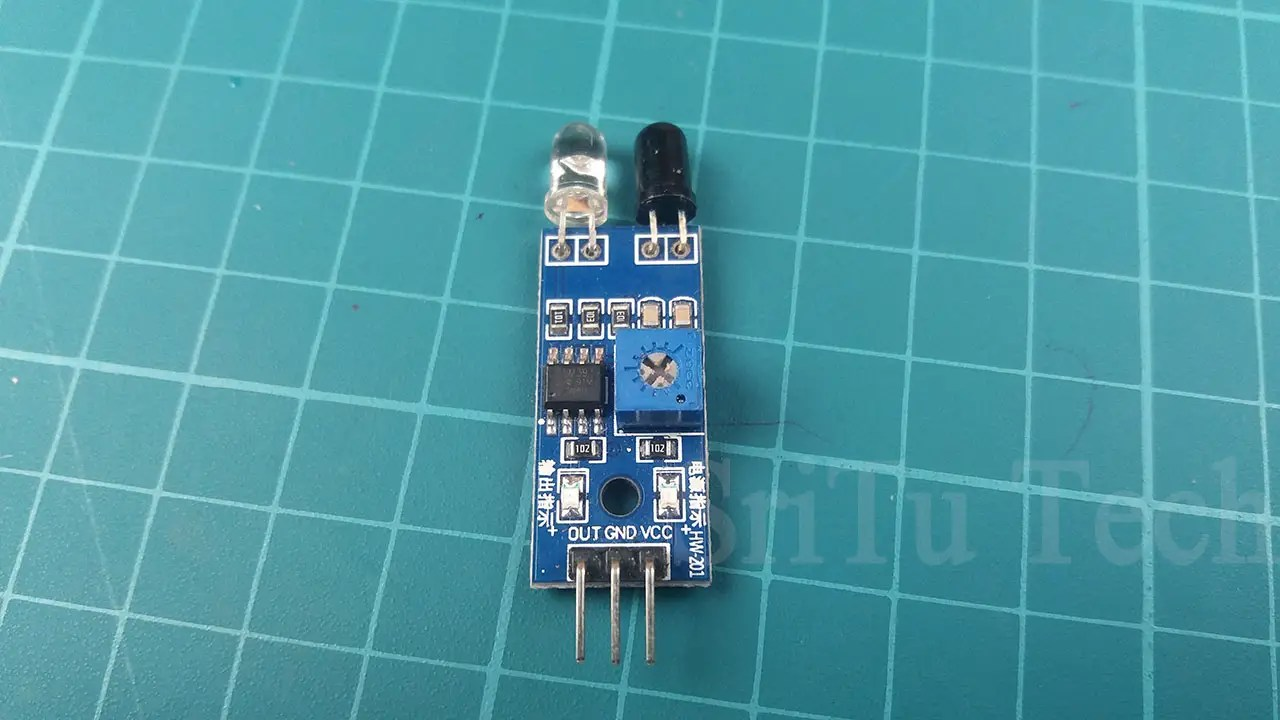With this sensor, we can only get digital signals.
LED bulbThis is used to test a barrier near the sensor.Use this component to assemble the circuit.
Jumper wiresThis project using five MALE to MALE jumper wires.
Step 2

Step 3

Connect these components to your Arduino board using the jumper wires. Do it according to the circuit diagram below.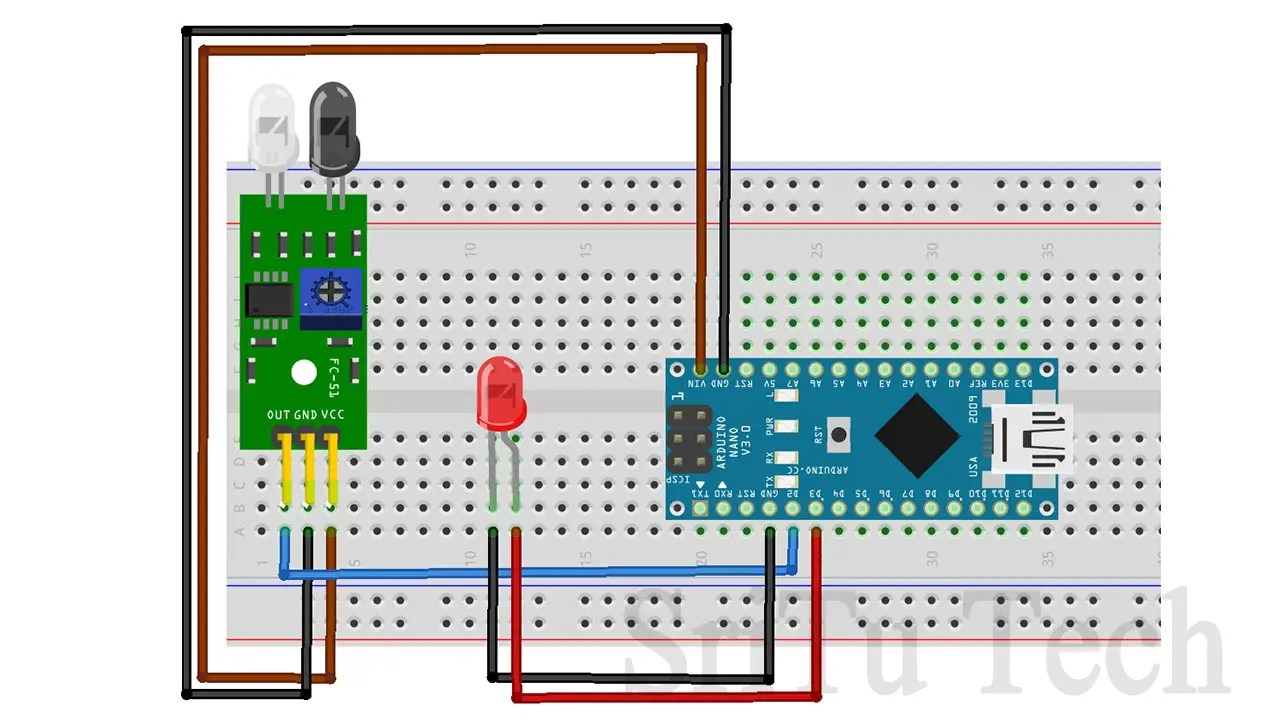Connect the sensor OUT pin to the Arduino board D2 pin and connect the LED anode pin to the D3 pin.

### Step 4

Let’s look at the code below.
``````void setup() {
pinMode(2, INPUT);//define arduino pin
pinMode(3, OUTPUT);//define arduino pin
Serial.begin(9600);//enable serial monitor
}
void loop() {
bool value = digitalRead(2);//get value and save it boolean veriable
if (value == 1) { //check condition
Serial.println("ON");//print serial monitor ON
digitalWrite(3,HIGH);//LED on
} else {
Serial.println("OFF");//print serial monitor OFF
digitalWrite(3,LOW);//LED off
}
}``````
This code sets 2 pins as input pins and 3 pins as output pins.
void setup() {
pinMode(2, INPUT);//define arduino pin
pinMode(3, OUTPUT);//define arduino pin
Serial.begin(9600);//enable serial monitor
}

Takes the digital value and puts it into the Boolean variable. The Boolean variable is named ‘value’.
bool value = digitalRead(2);//get value and save it boolean veriable

This value is checked using the IF condition. When the digital value is 1, the LED  bulb turns on and when the digital value is 0, the LED bulb turns off.
if (value == 1) { //check condition
Serial.println(“ON”);//print serial monitor ON
digitalWrite(3,HIGH);//LED on
} else {
Serial.println(“OFF”);//print serial monitor OFF
digitalWrite(3,LOW);//LED off
}
Step 5
Select board and port.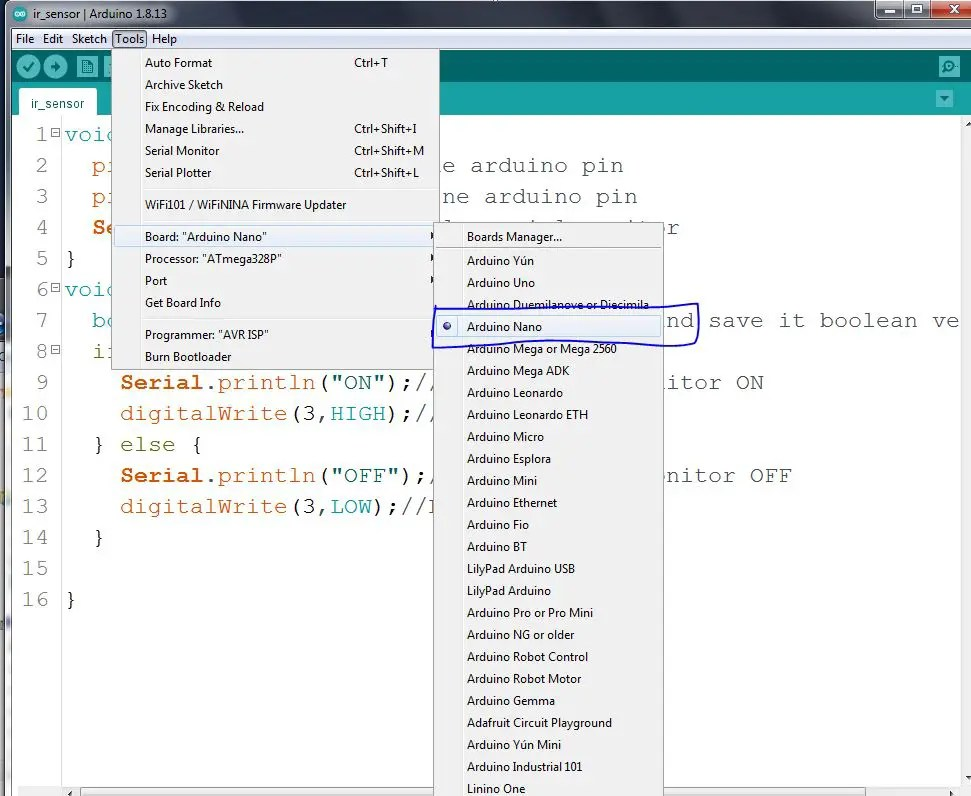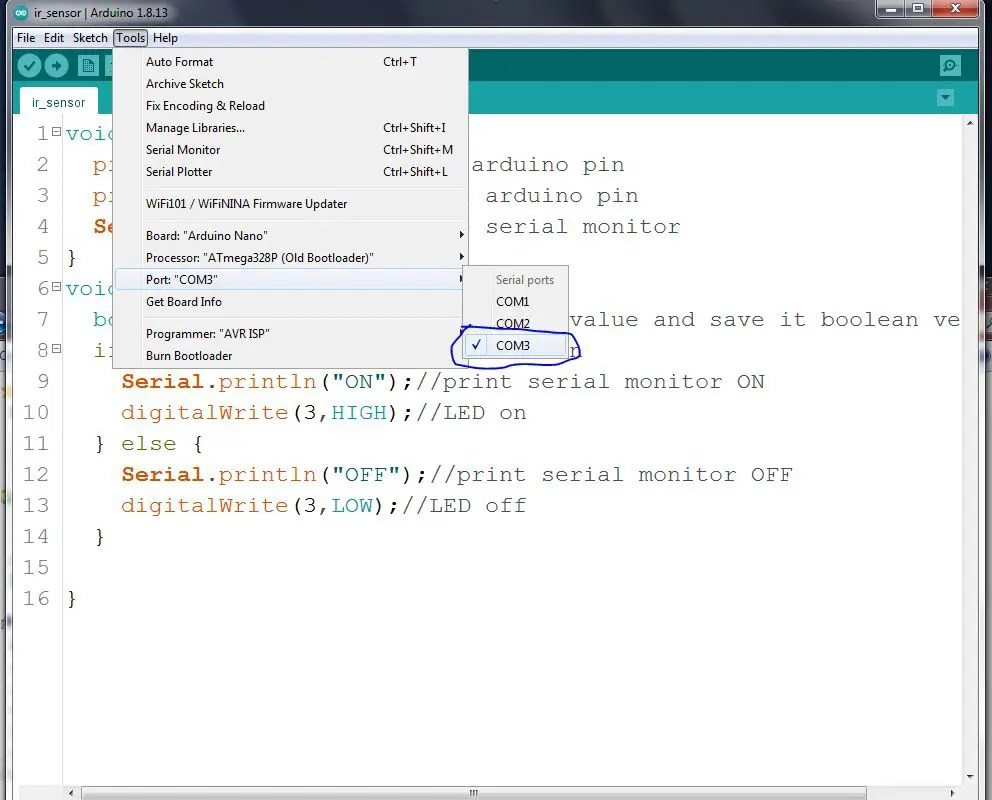#### Step 6

OK. now upload this code to the Arduino board.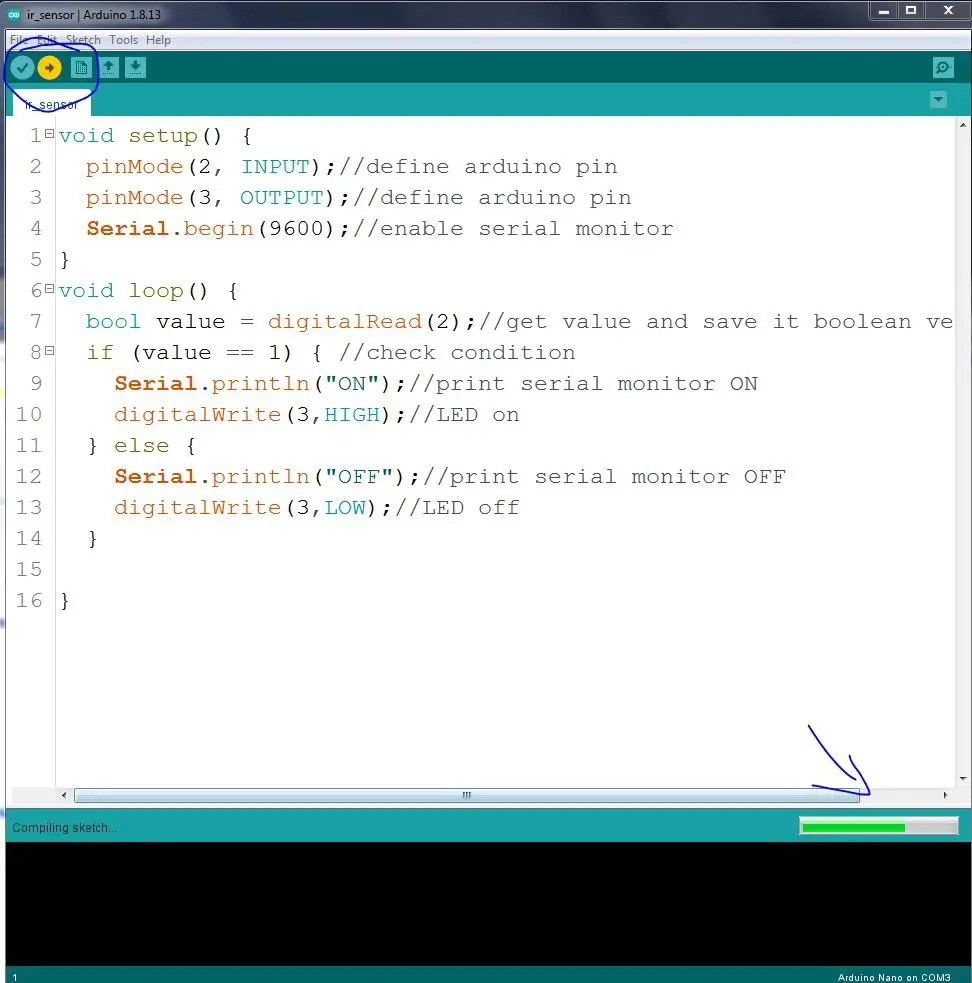So, now you can test this sensor. The full video guide is given below.

### 3rd sensor type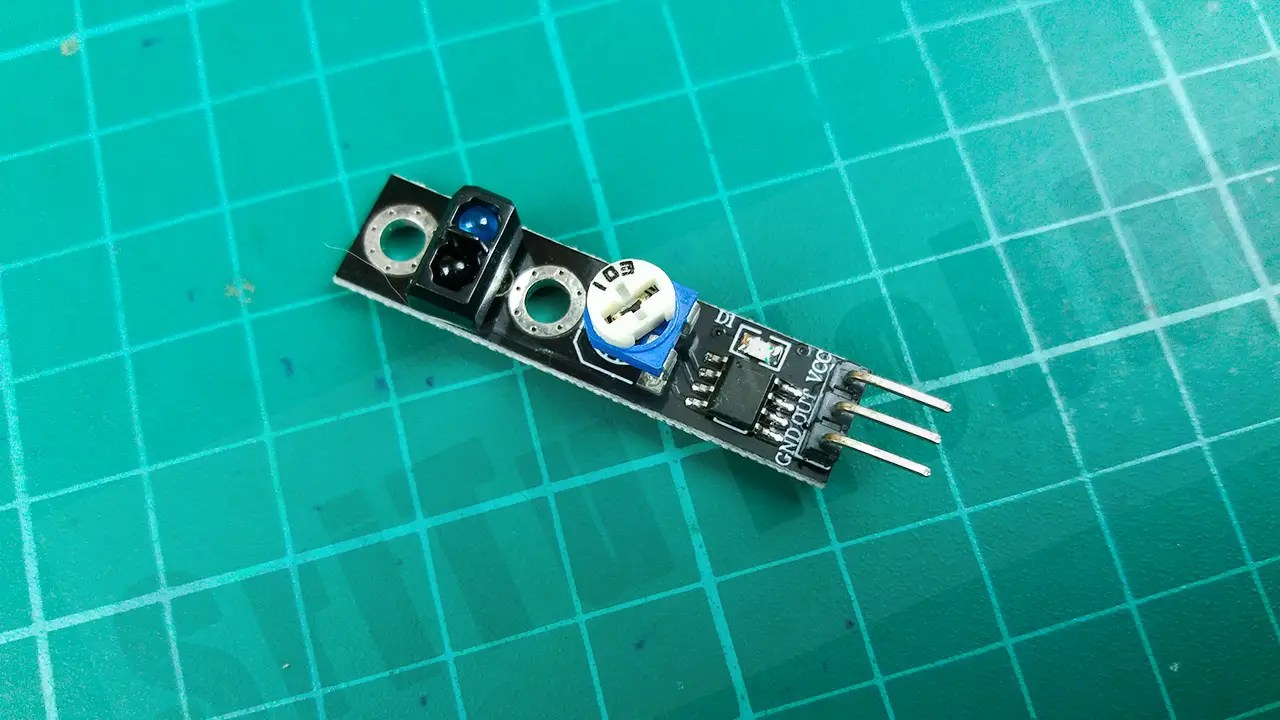This sensor includes three pins. Also, we can get a digital value through this sensor. This sensor can also be used to detect black and white as well as obstacles. We can control the sensing range using the potentiometer on this sensor.

#### PIN diagram of this sensorSo, step by step we will learn how to connect this sensor with Arduino. For that, the required components are given below.

### Step 1

Firstly, identify these components

#### Arduino Nano board.#### LED.180-ohm resistor.#### Buzzer.#### Jumper wires.#### Step 2

Secondly, connect these components. For that, use the circuit diagram below.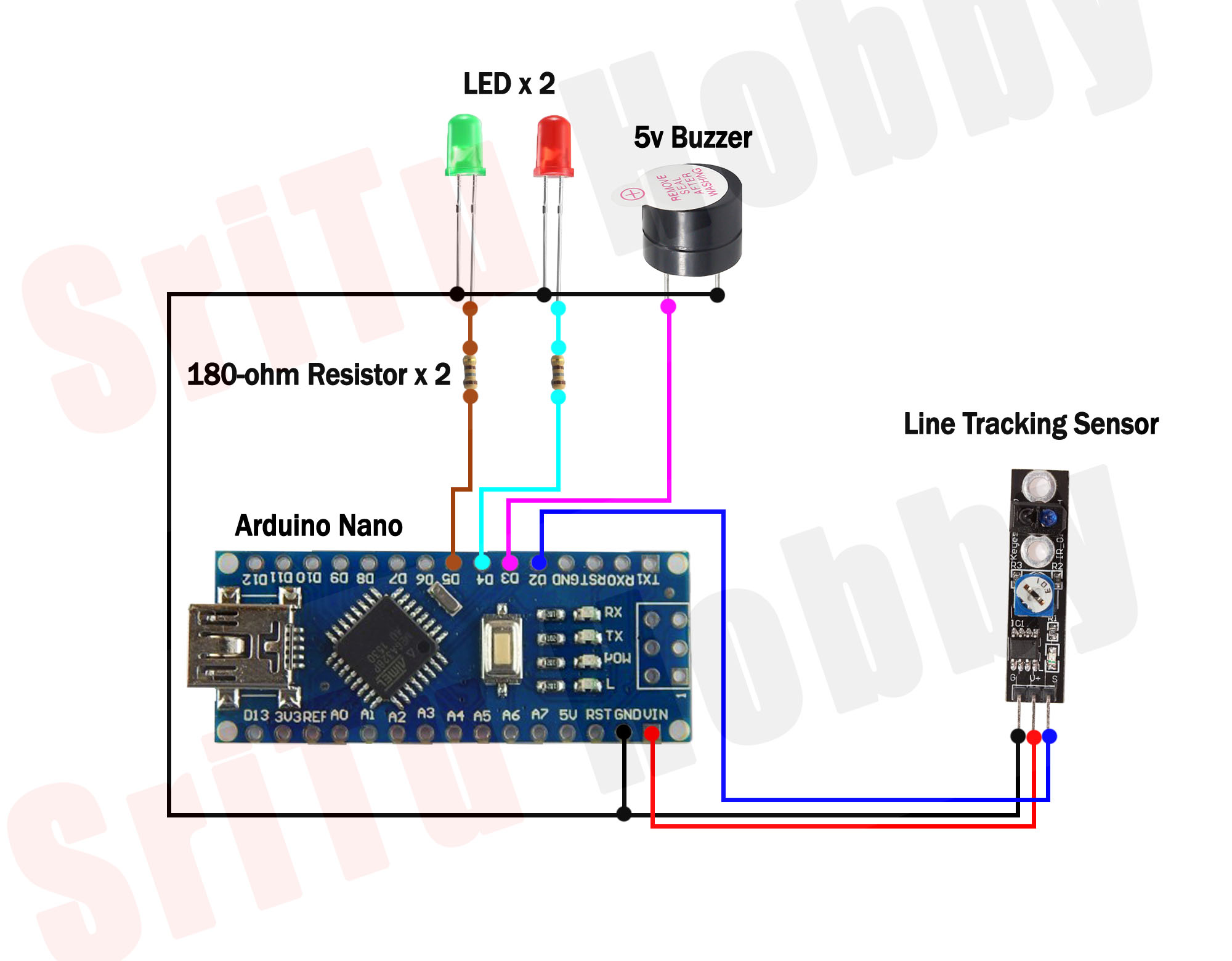### Step 3

Thirdly, we will create the required program for this. It is as follows.
``````/*Line tracking sensor tutorial.
*This code created by the SriTu Hobby team.
* https://srituhobby.com
*/

#define Sensor 2
#define Buzzer 3
#define Red 4
#define Green 5

void setup() {
Serial.begin(9600);
pinMode(Sensor, INPUT);
pinMode(Buzzer, OUTPUT);
pinMode(Green, OUTPUT);
pinMode(Red, OUTPUT);
}
void loop() {
Serial.println(value);

if (value == 0) {
digitalWrite(Buzzer, HIGH);
digitalWrite(Green, HIGH);
digitalWrite(Red, LOW);
} else if (value == 1) {
digitalWrite(Buzzer, LOW);
digitalWrite(Green, LOW);
digitalWrite(Red, HIGH);
}
}``````

Code explanation

Firstly, this code defines the sensor, LED, and buzzer-connected pins.
#define Sensor 2
#define Buzzer 3
#define Red 4
#define Green 5

Secondly, this code sets the sensor pin as the input pin and the buzzer, LED pin as the output pin.
void setup() {
Serial.begin(9600);
pinMode(Sensor, INPUT);
pinMode(Buzzer, OUTPUT);
pinMode(Green, OUTPUT);
pinMode(Red, OUTPUT);
}
In the loop function, the sensor values are taken and put into the Boolean variable. After, these values are then checked using the IF condition. Then if the value is 0, the buzzer and green LED bulb will be activated. If the value is 1, the red LED bulb will be activated.
void loop() {
Serial.println(value);

if (value == 0) {
digitalWrite(Buzzer, HIGH);
digitalWrite(Green, HIGH);
digitalWrite(Red, LOW);
} else if (value == 1) {
digitalWrite(Buzzer, LOW);
digitalWrite(Green, LOW);
digitalWrite(Red, HIGH);
}
}

### Step 4

Lastly, select board and port. Afterward, upload this code.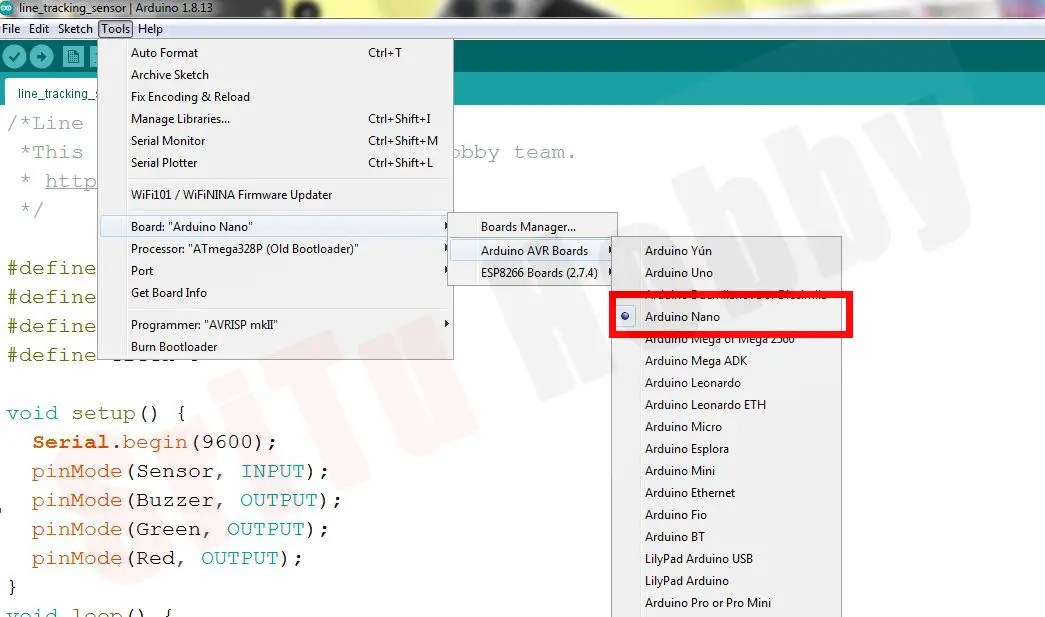OK, now you can test this sensor. For that, use a black and white surface. The full video guide is given below. So, we will meet in the next tutorial.
Shopping Cart
Scroll to Top